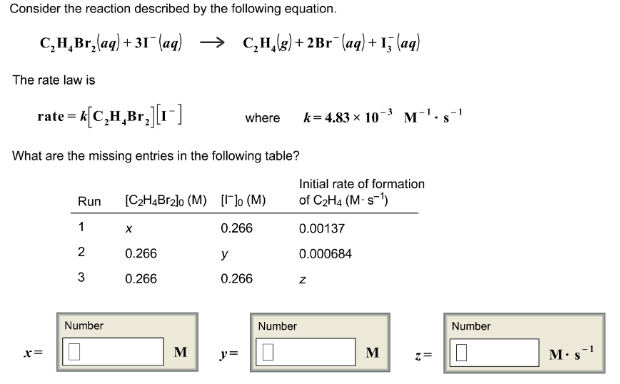# Problem: Consider the reaction described by the following equation.C2H4Br3 (aq) + 3I- (aq) → C2H4 (g) + 2Br- (aq) + I3- (aq) The rate law is rate = k[C2H4Br2][I-] where k = 4.83 x 10-3 M-1 • s-1 What are the missing entries in the following table?

###### FREE Expert Solution
95% (488 ratings)###### Problem Details

Consider the reaction described by the following equation.

C2H4Br3 (aq) + 3I- (aq) → C2H4 (g) + 2Br- (aq) + I3(aq)

The rate law is rate = k[C2H4Br2][I-] where k = 4.83 x 10-3 M-1 • s-1

What are the missing entries in the following table?